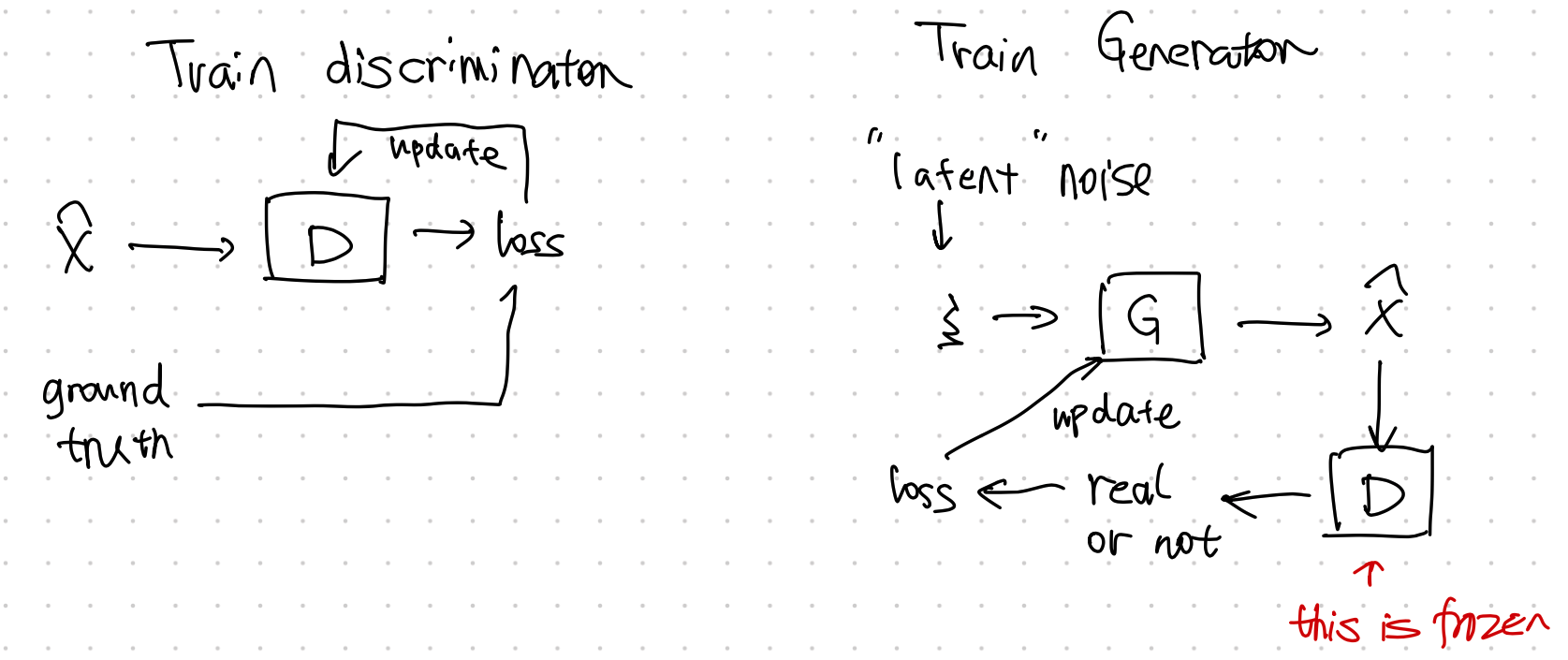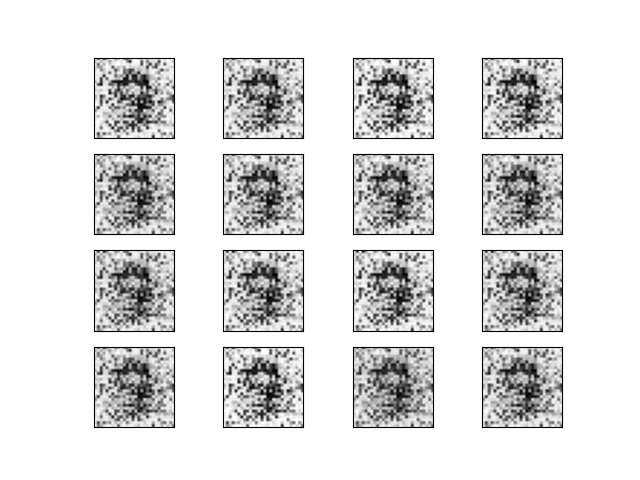# GAN¶

GAN is a generative neural sampler1. To train the sampler, the task of GAN is designed to generate features $$X$$ from a latent space $$\xi$$ and class labels $$Y$$,

$\xi, Y \to X.$

Many different formulations of GANs are proposed. As an introduction to this topic, we will discuss vanilla GAN in this section3.

## Theory¶

### The Minimax Game Loss¶

The minimax game is a game to "minimizing the possible loss for a worst case"2. In GAN, the game is to train the generator $${\color{red}G}$$ to fool the discriminator $${\color{green}D}$$ while minimizing the discrimination error of $${\color{green}D}$$.

Goodfellow prosed a loss3

$\begin{equation} \underset{{\color{red}G}}{\operatorname{min}}\underset{{\color{green}D}}{\operatorname{max}} V({\color{green}D}, {\color{red}G}) = \mathbb E_{x\sim p_{data}} \left[ \log {\color{green}D}(x) \right] + \mathbb E_{z\sim p_z} \left[ \log( 1- {\color{green}D}({\color{red}G}(z)) ) \right]. \end{equation}$

### Divergence¶

Goodfellow et al proved that the global minimum of such a setup is reached only and if only $$p_{G} = p_\text{data}$$. GAN is comparing the generated distribution to the data distribution, using the Jensen-Shannon divergence3,

$\operatorname{D}_{\text{JS}}(p_\text{data}\Vert p_{{\color{red}G}}) = \frac{1}{2}\left[ \operatorname{D}_\text{KL} \left( p_\text{data} \bigg\Vert \frac{p_\text{data} + p_{\color{red}G}}{2} \right) + \operatorname{D}_\text{KL} \left( p_{{\color{red}G}} \bigg\Vert \frac{p_\text{data} + p_{\color{red}G}}{2} \right) \right].$

Off by a Constant

The value function of GAN for fixed $$G$$ is slightly different from JS divergence3,

$\underset{G}{\operatorname{max}}V({\color{red}G},{\color{green}D}) = 2 \operatorname{D}_\text{JS}( p_\text{data} \Vert p_{\color{red}G} ) - \log 4.$

### Alternating Training¶

GAN training requires two stages,

• train discriminator $${\color{green}D}$$, and
• train generator $${\color{red}G}$$.## Code¶

We built a simple GAN using MNIST dataset.

The generated images looks quite close to hand writings.import matplotlib.pyplot as plt
import torch
from pathlib import Path
import torchvision
import torchvision.transforms as transforms
from loguru import logger
from torch import nn
import click

logger.debug(f"Setting device ...")
device = ""
if torch.cuda.is_available():
device = torch.device("cuda")
else:
device = torch.device("cpu")
logger.info(f"Device in use: {device}")

def plot_images(image_samples, target):
"""Plot a grid of images and save to a file."""

if not Path(target).parent.exists():
Path(target).parent.mkdir(parents=True)

for i in range(16):
ax = plt.subplot(4, 4, i + 1)
plt.imshow(image_samples[i].reshape(28, 28), cmap="gray_r")
plt.xticks([])
plt.yticks([])
plt.savefig(target)

"""Get MNIST data and built a dataloader for the dataset"""

transform = transforms.Compose(
[transforms.ToTensor(), transforms.Normalize((0.5,), (0.5,))]
)

train_set = torchvision.datasets.MNIST(
)

train_set, batch_size=batch_size, shuffle=True
)

if plot_samples:
plot_images(real_samples, target="assets/real_images/real_image_samples.png")

class Discriminator(nn.Module):
"""The discrimnator should take data that has the dimension of the image and spit out a probability"""
def __init__(self):
super().__init__()
self.model = nn.Sequential(
nn.Linear(784, 1024),
nn.ReLU(),
nn.Dropout(0.3),
nn.Linear(1024, 512),
nn.ReLU(),
nn.Dropout(0.3),
nn.Linear(512, 256),
nn.ReLU(),
nn.Dropout(0.3),
nn.Linear(256, 1),
nn.Sigmoid(),
)

def forward(self, x):
x = x.view(x.size(0), 784)
output = self.model(x)
return output

class Generator(nn.Module):
"""The generator should take in some noise data (a latent space data) and spit out an image.
We use the input noise as a trick to make the generator more general
"""
def __init__(self):
super().__init__()
self.model = nn.Sequential(
# nn.Linear(10, 100),
# nn.ReLU(),
nn.Linear(100, 256),
nn.ReLU(),
nn.Linear(256, 512),
nn.ReLU(),
nn.Linear(512, 1024),
nn.ReLU(),
nn.Linear(1024, 784),
nn.Tanh(),
)

def forward(self, x):
output = self.model(x)
output = output.view(x.size(0), 1, 28, 28)
return output

@click.command()
@click.option("--epochs", default=50, help="Number of epochs for the training")
@click.option("--learning_rate", "-lr", default=0.0001, help="Learning rate for the optimizer")
@click.option("--batch_size", default=32, help="Batch size")
@click.option("--data_dir", default="data/mnist", help="Directory for storing the dataset")
@click.option("--random_seed", "-rs", default=42, type=int, help="Random seed for the random generators")

latent_space_dim = 100

torch.manual_seed(random_seed)

# check the dtypes
logger.debug(
f"torch tensor dtype: {torch.tensor([1.2, 3]).dtype}"
)
# torch.set_default_dtype(torch.float64)
# logger.debug(
#     f"set torch tensor dtype to 64: {torch.tensor([1.2, 3]).dtype}"
# )

batch_size=batch_size,
data_dir=data_dir,
)

discriminator = Discriminator().to(device=device)
generator = Generator().to(device=device)

loss_function = nn.BCELoss()

discriminator.parameters(), lr=learning_rate
)

for epoch in range(epochs):
for n, (real_samples, mnist_labels) in enumerate(train_loader):
# We prepare some data for training the discriminator
# Here we will prepare both the generated data and the real data
real_samples = real_samples.to(device=device)
real_samples_labels = torch.ones((batch_size, 1)).to(device=device)
latent_space_samples = torch.randn((batch_size, latent_space_dim)).to(device=device)
# logger.debug(f"Latent space samples: {latent_space_samples}")
generated_samples = generator(latent_space_samples)
# logger.debug(f"Generated samples:{generated_samples}")
generated_samples_labels = torch.zeros((batch_size, 1)).to(device=device)
all_samples = torch.cat((real_samples, generated_samples))
all_samples_labels = torch.cat((real_samples_labels, generated_samples_labels))

# Training the discriminator
# The discrinimator is trained using the samples we generated above, i.e.
# the generated samples and the real images
output_discriminator = discriminator(all_samples)
loss_discriminator = loss_function(output_discriminator, all_samples_labels)
loss_discriminator.backward()
optimizer_discriminator.step()

# Generate some noise data for training the generator
#
latent_space_samples_generator = torch.randn((batch_size, latent_space_dim)).to(device=device)

# Training the generator using the training optimizer
generated_samples_generator = generator(latent_space_samples_generator)
output_discriminator_generated = discriminator(generated_samples_generator)
loss_generator = loss_function(
output_discriminator_generated, real_samples_labels
)
loss_generator.backward()
optimizer_generator.step()

# Show loss
if n == batch_size - 1:
print(f"Epoch: {epoch} Loss D.: {loss_discriminator}")
print(f"Epoch: {epoch} Loss G.: {loss_generator}")

logger.debug(f"Plotting for epoch: {epoch} ...")
latent_space_samples_epoch = torch.randn(batch_size, latent_space_dim).to(device=device)
generated_samples_epoch = generator(latent_space_samples_epoch)
generated_samples_epoch = generated_samples_epoch.cpu().detach()
plot_images(generated_samples_epoch, target=f"assets/generated_images/generated_image_samples_{epoch}.png")
logger.debug(f"Saved plots for epoch: {epoch}")

latent_space_samples = torch.randn(batch_size, latent_space_dim).to(device=device)
generated_samples = generator(latent_space_samples)

logger.debug(f"Plot generated images...")
generated_samples = generated_samples.cpu().detach()
plot_images(generated_samples, target="assets/generated_images/generated_image_samples.png")

if __name__ == "__main__":
main()


1. Nowozin S, Cseke B, Tomioka R. f-GAN: Training Generative Neural Samplers using Variational Divergence Minimization. arXiv [stat.ML]. 2016. Available: http://arxiv.org/abs/1606.00709

2. Contributors to Wikimedia projects. Minimax. In: Wikipedia [Internet]. 5 Aug 2021 [cited 6 Sep 2021]. Available: https://en.wikipedia.org/wiki/Minimax

3. Goodfellow IJ, Pouget-Abadie J, Mirza M, Xu B, Warde-Farley D, Ozair S, et al. Generative Adversarial Networks. arXiv [stat.ML]. 2014. Available: http://arxiv.org/abs/1406.2661

4. Liu X, Zhang F, Hou Z, Wang Z, Mian L, Zhang J, et al. Self-supervised Learning: Generative or Contrastive. arXiv [cs.LG]. 2020. Available: http://arxiv.org/abs/2006.08218

5. Arjovsky M, Chintala S, Bottou L. Wasserstein GAN. arXiv [stat.ML]. 2017. Available: http://arxiv.org/abs/1701.07875

Contributors: LM